• 行列式求偏导
千次阅读
2020-05-06 16:09:59

这里我们大致地复习一下偏导数，雅克比矩阵以及黑塞矩阵的定义和关系。

##### 导数向量与雅克比矩阵（Jacobi matrix）

函数的某个因变量对某个自变量求的导数即为它们关于函数的偏导数。当因变量为一元的情况下，各个自变量的偏导数组成了导数向量.当函数因变量为多元的情况下，函数的导数可由jacobi matrix来描述。E.g., 现有一函数可将m维的自变量映射到n维的因变量上，也就是说该函数由n个子函数构成 y 1 ( x 1 , . . . , x m ) , . . . , y n ( x 1 , . . . , x m ) y_1(x_1,...,x_m),...,y_n(x_1,...,x_m) .而jacobi matrix则由这些自变量和因变量之间的偏导数组成，构成一个n行m列的矩阵：
[ ∂ y 1 ∂ x 1 ⋯ ∂ y 1 ∂ x m ⋮ ⋱ ⋮ ∂ y n ∂ x 1 ⋯ ∂ y n ∂ x m ] (3) \left[ \begin{matrix} \frac{\partial y_1}{\partial x_1} & \cdots & \frac{\partial y_1}{\partial x_m} \\ \vdots & \ddots & \vdots \\ \frac{\partial y_n}{\partial x_1} & \cdots & \frac{\partial y_n}{\partial x_m} \end{matrix} \right] \tag{3}
这个Jacobi matrix可以写作 J F ( x 1 , . . . , x m ) J_F(x_1,...,x_m) ,或者是 ∂ ( y 1 , . . . , y n ) ∂ ( x 1 , . . . , x m ) \frac{\partial (y_1,...,y_n)}{\partial (x_1,...,x_m)}

##### 黑塞矩阵（Hessian matrix）

一句话来讲，Hessian matrix是多元函数（单因变量）的二阶偏导数组成的方阵，它也可以被理解为该函数的一阶导数向量的Jacobi matrix!当函数满足：
y = f ( x 1 , . . . , x m ) y = f(x_1,...,x_m) 该函数存在一阶偏导数向量：
[ ∂ y ∂ x 1 , . . . ∂ y ∂ x m ] [\frac{\partial y}{\partial x_1},...\frac{\partial y}{\partial x_m}] .若该函数存在二阶导数，则其Hessian matrix H ( f ) H(f) 为：
[ ∂ 2 y ∂ x 1 2 ⋯ ∂ 2 y ∂ x 1 ∂ x m ⋮ ⋱ ⋮ ∂ 2 y ∂ x m ∂ x 1 ⋯ ∂ 2 y ∂ x m 2 ] (3) \left[ \begin{matrix} \frac{\partial^2 y}{\partial x_1^2} & \cdots & \frac{\partial^2 y}{\partial x_1 \partial x_m} \\ \vdots & \ddots & \vdots \\ \frac{\partial^2 y}{\partial x_m \partial x_1} & \cdots & \frac{\partial^2 y}{\partial x_m^2} \end{matrix} \right] \tag{3}

更多相关内容
• 线性代数之行列式偏导 矩阵偏导 针对y或者f(x)是元素，x是矩阵的情况，则元素对矩阵的求导形式如下： 注:这里的 ​和矩阵x是同型（行数列数相同）的。 行列式性质 这里假定A是方阵，则有如下性质： 1 ...

# 线性代数之行列式偏导

## 矩阵偏导

针对y或者f(x)是元素，x是矩阵的情况，则元素对矩阵的求导形式如下：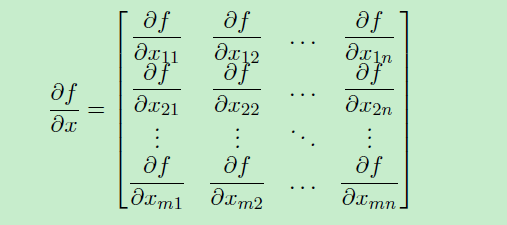:这里的​ 和矩阵x是同型（行数列数相同）的。

## 行列式性质

这里假定A是方阵，则有如下性质：

1 余子式是将行列式的第i行、第j列删除后组成的新行列式，一般用如下符号表示：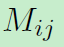比较一般的例子(M11)见：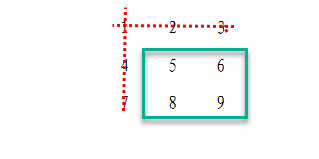2 对余子式求余因子，则称为代数余子式。表达式为：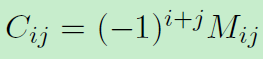3 行列式的值等于某一行(列)的各个元素与其对应的代数余子式的乘积之和，即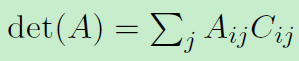4由行列式A的代数余子式Aij 的转置所构成的矩阵叫做伴随矩阵，其定义如下：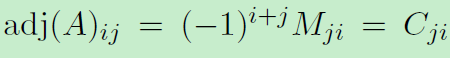即可简写为：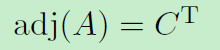，其中C为代数余子式。

5 对于非奇异（行列式不为0）的矩阵A，有如下性质：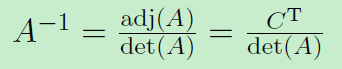这里由伴随矩阵的定义得：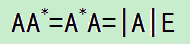，因为A可逆，则容易得上式(即中间式子右乘A-1)。

：这里的A*即是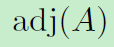## 行列式偏导

因为行列式是标量函数，所以之前关于标量函数的定义与性质都适应于行列式。

按照行列式分量展开的形式看，则有：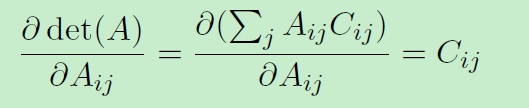针对整个行列式，则有: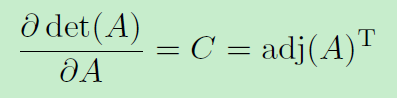：1 这里借助矩阵导数的概念：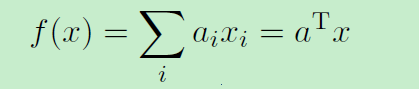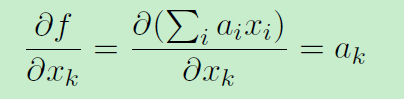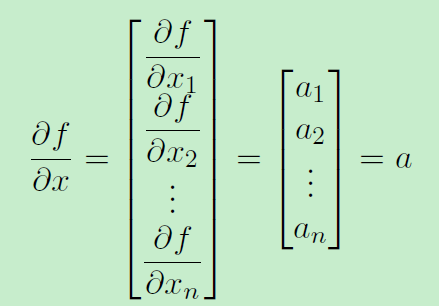2 伴随矩阵等于代数余子式矩阵的转置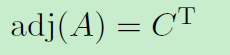## 行列式偏导与矩阵逆

接行列式偏导的定义，针对行列式A是非奇异(行列式不为0)的情况，则可以进一步转换：

1 将伴随矩阵转换为行列式和矩阵逆的乘积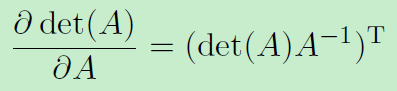2 再结合常数乘矩阵逆的性质，即可将常数提到外面，最终得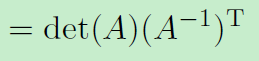展开全文机器学习
• ## 对范数求偏导数

千次阅读 2017-09-24 21:14:08
AHA^H表示Hermitian矩阵(A的共轭转置矩阵A∗==A)基础（1）迹（Trace）eig(A)表示A的特征值（2）行列式（Determinant）（3）特例2*2矩阵以上是摘自：The Matrix Cookbook 也可参考维基百科：Matrix calculusL1范数的...

首先介绍点基础知识，另一方面也算是巩固下：
A1 $A^{-1}$表示A的逆矩阵;
AT $A^T$表示A的转置;
AH $A^H$表示Hermitian矩阵(A的共轭转置矩阵A∗==A)

### 基础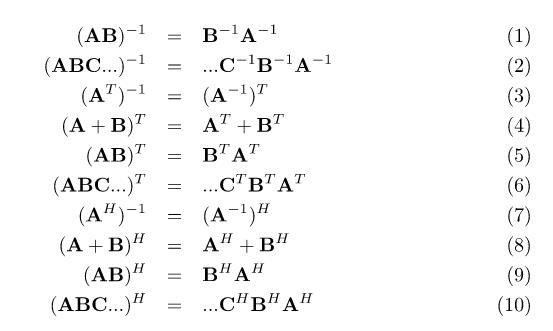### （1）迹（Trace）

eig(A)表示A的特征值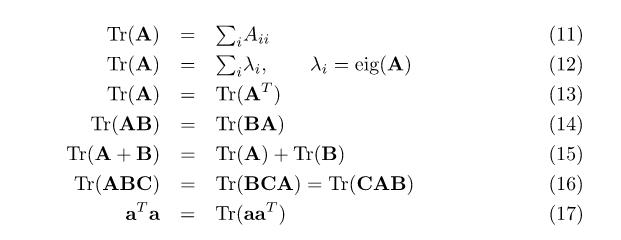### （2）行列式（Determinant）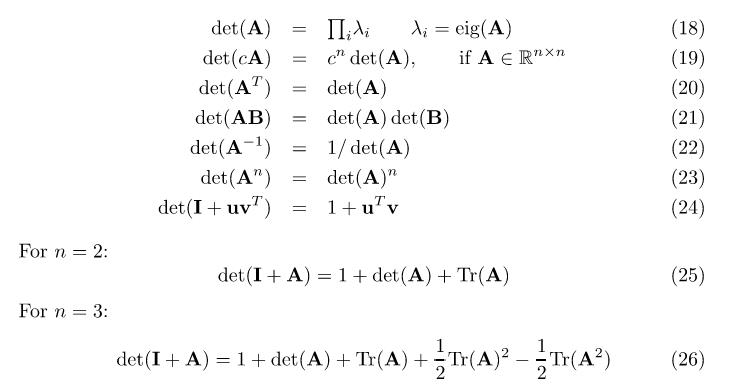### （3）特例2*2矩阵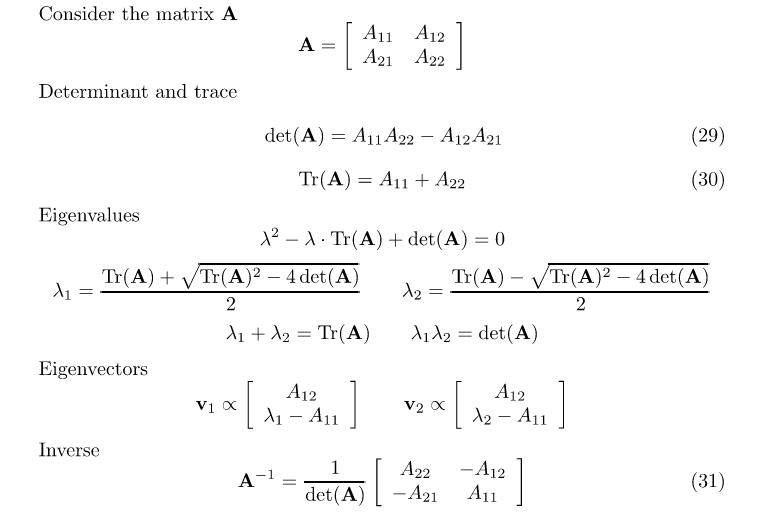以上是摘自：The Matrix Cookbook
也可参考维基百科：Matrix calculus

### L1范数的次微分

L1范数不可微。但是存在次梯度，即是次微分的
L1范数的次梯度如下：

x||x||1=sign(x)

其中sign(x) 表示如下：
sign(x)=+11[1,1]xi>0xi<0xi=0

L1 $L_1$范数：
||X||1=|x1|+|x2|++|xn|

例如： x=(3,2,5)T $x=(3,2,-5)^T$
故其梯度为：sign(x)=(1,1,-1)

### L2范数的微分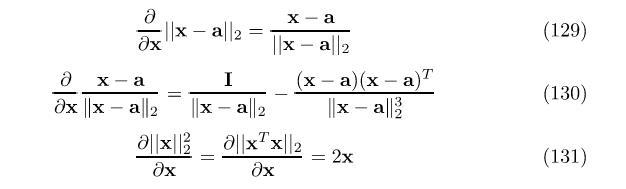例如：求解下面函数的偏导数：

f(W)=12i,jϵSγi,j||wTiXwTjX||22

得：
f(W)wi=i,jϵsγi,j(wTiXwTjX)(wTiXwTjX)wi=i,jϵsγi,j(wTiXwTjX)XT=i,jϵsγi,j(wTiwTj)(XXT)

注意这里得到的是行向量的形式，因此还需要对其进行转置

以上的推倒是基于上图公式得到。。。

展开全文matrix
• 雅可比矩阵和行列式(Jacobian Matrix and Determinant) 给定一个将 $n$ 维输入向量 $\boldsymbol{x}$ 映射到 $m$ 维的函数 $\boldsymbol{f} : \mathbb{R}^{n} \mapsto \mathbb{R}^{m}$，该函数的所有一阶偏导数组成...

在本文中，我们将深入探讨第三种生成模型：基于流的生成模型(Flow-based generative models)。与GAN和VAE不同，这种模型显式地学习输入数据的概率密度函数。

截至目前，我已介绍了两种生成模型，GAN和VAE。它们都不能显式地计算出真实数据的概率密度函数 $p(\boldsymbol{x}),\boldsymbol{x} \in \mathcal{D}$，因为这真的很难！以含有隐变量的生成模型为例，$p(\boldsymbol{x})=\int p(\boldsymbol{x} | \boldsymbol{z}) p(\boldsymbol{z}) d \boldsymbol{z}$ 几乎不可能被算出，因为遍历所有隐变量 $\boldsymbol{z}$ 的值是不可能的。

在normalizing flow的帮助下，基于流的生成模型解决了这个困难的问题。对于 $p(\boldsymbol{x})$ 的良好估计使得许多下游任务能被高效完成：数据生成(data generation)、密度估计(density estimation)、推理隐变量(infer latent variables)等。

生成模型的种类(Type of Generative Models)

以下是GAN、VAE和flow-based models的主要区别：GAN：生成式对抗网络(GAN)使用一种十分聪明的方式将数据生成这个无监督问题转化为一个有监督问题。判别模型学习如何将真实数据从生成模型生成的假样例中区分出来，两个模型以一种minmax游戏的方式进行训练。

VAE：变分自动编码器(VAE)通过最大化证据下界(ELBO)来隐式地最优化数据的对数似然。

Flow-based generative models：基于流的生成模型由一系列可逆变换(invertible transformations)组成。不像其他两种模型，基于流的模型显式地学习数据分布 $p(\boldsymbol{x})$，因此其损失函数就是简单的负对数似然函数。线性代数基础(Linear Algebra Basics Recap)

在更加深入地了解基于流的生成模型之前，我们应该理解两个关键概念：雅可比矩阵行列式和变量变换定理。

雅可比矩阵和行列式(Jacobian Matrix and Determinant)

给定一个将 $n$ 维输入向量 $\boldsymbol{x}$ 映射到 $m$ 维的函数 $\boldsymbol{f} : \mathbb{R}^{n} \mapsto \mathbb{R}^{m}$，该函数的所有一阶偏导数组成的 $m \times n$ 矩阵称为雅可比矩阵 $\boldsymbol{J}$：

$$\boldsymbol{J}=\left[\begin{array}{ccc}{\frac{\partial f_{1}}{\partial x_{1}}} & {\cdots} & {\frac{\partial f_{1}}{\partial x_{n}}} \\ {\vdots} & {\ddots} & {\vdots} \\ {\frac{\partial f_{m}}{\partial x_{1}}} & {\cdots} & {\frac{\partial f_{m}}{\partial x_{n}}}\end{array}\right]$$

行列式是由方阵的所有元素计算得到的一个实数。行列式的绝对值可以视为方阵列向量(或行向量)所张成的平行多面体的体积。

$n\times n$ 矩阵 $M$ 的行列式为：

$$\operatorname{det} M=\operatorname{det}\left[\begin{array}{cccc}{a_{11}} & {a_{12}} & {\dots} & {a_{1 n}} \\ {a_{21}} & {a_{22}} & {\dots} & {a_{2 n}} \\ {\vdots} & {\vdots} & {} & {\vdots} \\ {a_{n 1}} & {a_{n 2}} & {\dots} & {a_{n n}}\end{array}\right]=\sum_{j_{1} j_{2} \dots j_{n}}(-1)^{\tau\left(j_{12} \dots j_{n}\right)} a_{1 j_{1}} a_{2 j_{2}} \dots a_{n j_{n}}$$

方阵 $M$ 的行列式决定了其是否可逆：如果 $\operatorname{det}(M)=0$ 则 $M$ 不可逆，反之则可逆。

矩阵乘积的行列式等于矩阵行列式的乘积：

$$\operatorname{det}(A B)=\operatorname{det}(A) \operatorname{det}(B)$$

变量变换定理(Change of Variable Theorem)

让我们在概率密度估计问题的背景下复习一下变量变换定理，首先以单变量的情况为例。

给定一个随机变量 $z$，并已知其概率密度函数 $z \sim \pi(z)$。我们使用1-1映射 $x=f(z)$ 构造一个新的随机变量 $x$，映射函数 $f$ 是可逆的，故 $z=f^{-1}(x)$ 。现在的问题是：如何推断出新的变量 $x$ 的概率密度函数 $p(x)$？

$$\int p(x) d x=\int \pi(z) d z=1$$

$$p(x)=\pi(z)\left|\frac{d z}{d x}\right|=\pi\left(f^{-1}(x)\right)\left|\frac{d f^{-1}}{d x}\right|=\pi\left(f^{-1}(x)\right)\left|\left(f^{-1}\right)^{\prime}(x)\right|$$

根据定义，积分 $\int \pi(z) d z$ 是无数个宽度为无穷小 $\Delta z$ 的长方形的面积之和。这种长方形在 $z$ 处的高度为概率密度函数 $\pi(z)$ 的值。当我们替换变量时，由 $z=f^{-1}(x)$ 有 $\frac{\Delta z}{\Delta x}=\left(f^{-1}(x)\right)^{\prime}$，即 $\Delta z=\left(f^{-1}(x)\right)^{\prime} \Delta x$。

多变量的版本具有相似的形式：

$$\boldsymbol{z} \sim \pi(\boldsymbol{z}), \boldsymbol{x}=f(\boldsymbol{z}), \boldsymbol{z}=f^{-1}(\boldsymbol{x})$$

$$p(\boldsymbol{x})=\pi(\boldsymbol{z})\left|\operatorname{det} \frac{d \boldsymbol{z}}{d \boldsymbol{x}}\right|=\pi\left(f^{-1}(\boldsymbol{x})\right)\left|\operatorname{det} \frac{d f^{-1}}{d \boldsymbol{x}}\right|$$

其中 $\operatorname{det} \frac{\partial f}{\partial \boldsymbol{z}}$ 是函数 $f$ 的雅可比行列式。

什么是标准化流？(What is Normalizing Flows?)

密度估计在许多机器学习问题中都有应用，但它十分困难。例如，由于我们在深度学习模型中需要进行反向传播，因此后验概率分布 $p(\boldsymbol{z} | \boldsymbol{x}))$ 应该足够简单，以便进行求导。这也是高斯分布在隐变量生成模型中被广泛应用的原因，即使大多数现实世界的分布要远比高斯分布复杂。

标准化流(Normalizing Flow)模型的提出就是为了获得更好、更强大的近似概率分布的能力。标准化流通过一系列可逆变换函数将一个简单的分布转化为一个复杂的分布。在一串变换中，我们根据变量变换定理重复地进行变量替换，并最终得到目标变量的概率分布。如上图所示：

$$\boldsymbol{Z}_{i-1} \sim p_{i-1}\left(\boldsymbol{Z}_{i-1}\right)$$

$$\boldsymbol{z}_{i}=f_{i}\left(\boldsymbol{z}_{i-1}\right), \text { thus } \boldsymbol{z}_{i-1}=f_{i}^{-1}\left(\boldsymbol{z}_{i}\right)$$

$$p_{i}\left(\boldsymbol{z}_{i}\right)=p_{i-1}\left(f_{i}^{-1}\left(\boldsymbol{z}_{i}\right)\right)\left|\operatorname{det} \frac{d f_{i}^{-1}}{d \boldsymbol{z}_{i}}\right|$$

接下来，让我们把该公式转化为 $\boldsymbol{z}_i$ 的函数，以便根据初始分布进行推理。

\begin{aligned} p_{i}\left(\boldsymbol{z}_{i}\right)&=p_{i-1}\left(f_{i}^{-1}\left(\boldsymbol{z}_{i}\right)\right)\left|\operatorname{det} \frac{d f_{i}^{-1}}{d \boldsymbol{z}_{i}}\right|\\ &=p_{i-1}\left(\boldsymbol{z}_{i-1}\right)\left|\operatorname{det}\left(\frac{d f_{i}}{d \boldsymbol{z}_{i-1}}\right)^{-1}\right|\\ &=p_{i-1}\left(\boldsymbol{z}_{i-1}\right)\left|\operatorname{det} \frac{d f_{i}}{d \boldsymbol{z}_{i-1}}\right|^{-1} \end{aligned}

上面的过程用到了以下数学定理。反函数定理

如果 $y=f(x),x=f^{-1}(y)$，则有：

$$\frac{d f^{-1}(y)}{d y}=\frac{d x}{d y}=\left(\frac{d y}{d x}\right)^{-1}=\left(\frac{d f(x)}{d x}\right)^{-1}$$可逆函数的雅可比行列式

可逆矩阵的逆的行列式等于该矩阵行列式的逆：

$$\operatorname{det}\left(M^{-1}\right)=(\operatorname{det}(M))^{-1}$$

这是因为

$$\operatorname{det}(M) \operatorname{det}\left(M^{-1}\right)=\operatorname{det}\left(M \cdot M^{-1}\right)=\operatorname{det}(I)=1$$

给定一系列这样的概率密度函数，并且已知每对相邻变量的关系，我们可以将公式一步一步进行扩展，直至追溯到初始分布 $\boldsymbol{z}_{0}$。

$$\boldsymbol{x}=\boldsymbol{z}_{K}=f_{K} \circ f_{K-1} \circ \cdots \circ f_{1}\left(\boldsymbol{z}_{0}\right)$$

\begin{aligned} \log p(\boldsymbol{x})=\log \pi_{K}\left(\boldsymbol{z}_{K}\right)&=\log \pi_{K-1}\left(\boldsymbol{z}_{K-1}\right)-\log \left|\operatorname{det} \frac{d f_{K}}{d \boldsymbol{z}_{K-1}}\right|\\ &=\log \pi_{K-2}\left(\boldsymbol{z}_{K-2}\right)-\log \left|\operatorname{det} \frac{d f_{K-1}}{d \boldsymbol{z}_{K-2}}\right|-\log \left|\operatorname{det} \frac{d f_{K}}{d \boldsymbol{z}_{K-1}}\right|\\ &=\ldots\\ &=\log \pi_{0}\left(\boldsymbol{z}_{0}\right)-\sum_{i=1}^{K} \log \left|\operatorname{det} \frac{d f_{i}}{d \boldsymbol{z}_{i-1}}\right| \end{aligned}

随机变量 $\boldsymbol{z}_{i}=f_{i}\left(\boldsymbol{z}_{i-1}\right)$ 穿过的路径被称为流(flow)，而连续分布 $\pi_{i}$ 组成的链被称为标准化流(normalizing flow)。出于计算的需求，变换函数 $f_i$ 应满足两个性质：容易求逆；

雅可比行列式容易计算。

标准化流模型(Models with Normalizing Flows)

当我们的工具箱内有了标准化流之后，输入数据的对数似然 $p(\boldsymbol{x})$ 就很容易处理了。基于流的生成模型的训练目标就是数据集 $\mathcal{D}$ 上的简单的负对数似然函数：

$$\mathcal{L}(\mathcal{D})=-\frac{1}{|\mathcal{D}|} \sum_{\boldsymbol{x} \in \mathcal{D}} \log p(\boldsymbol{x})$$

RealNVP

RealNVP(Real-valued Non-Volume Preserving)模型通过堆叠一系列可逆双射变换函数实现了标准化流。在每个被称为“仿射连接层”的双射 $f: \boldsymbol{x} \mapsto \boldsymbol{y}$ 中，输入向量(假设有 $D$ 维)的维度被分为两个部分：前 $d$ 个维度保持不变；

$d+1$ 到 $D$ 维进行一个仿射变换(放缩和平移，scale-and-shift)，并且放缩和平移的参数都是前 $d$ 个维度的函数。

\begin{aligned} \boldsymbol{y}_{1 : d} &=\boldsymbol{x}_{1 : d} \\ \boldsymbol{y}_{d+1 : D} &=\boldsymbol{x}_{d+1 : D} \odot \exp \left(s\left(\boldsymbol{x}_{1 : d}\right)\right)+t\left(\boldsymbol{x}_{1 : d}\right) \end{aligned}

其中 $s( .)$ 和 $t( .)$ 分别是放缩和平移函数，都进行映射 $\mathbb{R}^{d} \mapsto \mathbb{R}^{D-d}$。$\odot$ 运算表示对应元素相乘。

现在让我们来检查一下这种变换是否满足上文提到的两种性质。

条件1：容易求逆

显然该变换函数的逆很容易求出：

$$\left\{\begin{array}{l}{\boldsymbol{y}_{1 : d}=\boldsymbol{x}_{1 : d}} \\ {\boldsymbol{y}_{d+1 : D}=\boldsymbol{x}_{d+1 : D} \odot \exp \left(s\left(\boldsymbol{x}_{1 : d}\right)\right)+t\left(\boldsymbol{x}_{1 : d}\right)}\end{array} \Leftrightarrow\left\{\begin{array}{l}{\boldsymbol{x}_{1 : d}=\boldsymbol{y}_{1 : d}} \\ {\boldsymbol{x}_{d+1 : D}=\left(\boldsymbol{y}_{d+1 : D}-t\left(\boldsymbol{y}_{1 : d}\right)\right) \odot \exp \left(-s\left(\boldsymbol{y}_{1 : d}\right)\right)}\end{array}\right.\right.$$

条件2：雅可比行列式容易计算

该变换函数的雅可比行列式很容易求出，因为它的雅可比矩阵是一个下三角矩阵：

$$\boldsymbol{J}=\left[\begin{array}{cc}{\mathbb{I}_{d}} & {\boldsymbol{0}_{d \times(D-d)}} \\ {\frac{\partial \boldsymbol{y}_{d+1 : D}}{\partial \boldsymbol{x}_{1 : d}}} & {\operatorname{diag}\left(\exp \left(s\left(\boldsymbol{x}_{1 : d}\right)\right)\right)}\end{array}\right]$$

因此其雅可比行列式就是对角线元素的乘积：

$$\operatorname{det}(\boldsymbol{J})=\prod_{j=1}^{D-d} \exp \left(s\left(\boldsymbol{x}_{1 : d}\right)\right)_{j}=\exp \left(\sum_{j=1}^{D-d} s\left(\boldsymbol{x}_{1 : d}\right)_{j}\right)$$

看起来仿射连接层对于建立标准化流来说十分完美。除此之外，由于计算 $f^{-1}$ 不需要计算 $s$ 或 $t$ 的逆，并且计算雅可比行列式不需要计算 $s$ 或 $t$ 的雅可比行列式，因此这两个函数可以是任意复杂的，都可以用神经网络来表示。

在一个仿射连接层中，一些维度(信道)保持不变。为了保证所有输入都有机会被改变，模型在每一层中反转顺序。使用这种模式，在一个层中保持不变的单元在下一层中必被改变。批量归一化(batch normalization)操作被发现有助于模型的训练。

NICE

NICE(Non-linear Independent Component Estimation)模型是REALNVP的前作。NICE中的变换操作时不含有放缩操作的仿射连接层，被称为“加和连接层”(additive coupling layer)。

$$\left\{\begin{array}{l}{\boldsymbol{y}_{1 : d}=\boldsymbol{x}_{1 : d}} \\ {\boldsymbol{y}_{d+1 : D}=\boldsymbol{x}_{d+1 : D}+m\left(\boldsymbol{x}_{1 : d}\right)}\end{array} \Leftrightarrow\left\{\begin{array}{l}{\boldsymbol{x}_{1 : d}=\boldsymbol{y}_{1 : d}} \\ {\boldsymbol{x}_{d+1 : D}=\boldsymbol{y}_{d+1 : D}-m\left(\boldsymbol{y}_{1 : d}\right)}\end{array}\right.\right.$$

Glow

Glow模型拓展了之前的可能生成模型NICE和RealNVP，并将信道上的反向排列操作替换为可逆的1×1卷积。Glow中每一步流操作包含3个子步骤。

步骤1：Activation normalization(简写为actnorm)

该步骤使用一个放缩参数和一个偏移参数对每一个信道进行仿射变换，这与batch normalization类似，但在大小为1的mini-batch上工作。这两个参数是可以训练的，但由于具有初始化的值，因此数据的第一个minibatch在经过actnorm后均值为0，标准差为1。

步骤2：可逆 $1×1$ 卷积

在RealNVP流中，新到的顺序被反转，因此所有的数据维度都有机会被改变。而输入信道数和输出信道数相同的 $1×1$ 卷积可以进行任意顺序的信道排列操作。

也就是说，我们对 $h \times w \times c$ 维的输入张量 $\boldsymbol{h}$ 使用 $c\times c$ 权重矩阵 $\boldsymbol{w}$ 进行可逆的 $1\times1$ 卷积操作，输出为一个 $h \times w \times c$ 维的张量，表示为 $f=\operatorname{conv} 2 \mathrm{d}(\boldsymbol{h} ; \boldsymbol{W})$。为了应用变量变换定理，我们需要计算雅可比行列式 $|\operatorname{det} \partial f / \partial \boldsymbol{h}|$。

$1\times 1$ 卷积地输入和输出都可以被视为一个大小为 $h\times w$ 维的矩阵。输入张量 $\boldsymbol{h}$ 中的每个entry $\boldsymbol{x}_{i j}(i=1, \ldots, h, j=1, \ldots, w)$ 是一个 $c$ 信道的向量，每个entry分别与权重矩阵 $\boldsymbol{W}$ 相乘来获得输出矩阵中的对应entry。每个entry的导数为 $\partial \boldsymbol{x}_{i j} \boldsymbol{W} / \partial \boldsymbol{x}_{i j}=\boldsymbol{W}$，共有 $h\times w$个这样的entry，因此有：

$$\log \left|\operatorname{det} \frac{\partial \operatorname{conv} 2 \mathrm{d}(\boldsymbol{h} ; \boldsymbol{W})}{\partial \boldsymbol{h}}\right|=\log \left(|\operatorname{det} \boldsymbol{W}|^{h \cdot w} |\right)=h \cdot w \cdot \log |\operatorname{det} \boldsymbol{W}|$$

逆向的 $1\times 1$ 卷积依赖于逆矩阵 $\boldsymbol{W}^{-1}$。由于权重矩阵 $\boldsymbol{W}$ 相对来说规模较小，因此计算其行列式和逆矩阵的计算量仍在可控范围内。

步骤3：仿射连接层

这一部分的设计与RealNVP相同。展开全文• 在向量分析中，雅可比矩阵是函数的一阶偏导数以一定方式排列成的矩阵，其行列式称为雅可比行列式。 在代数几何中，代数曲线的雅可比行列式表示雅可比簇：伴随该曲线的一个代数群，曲线可以嵌入其中。 假设某函数从 ...
• ## jacobi 矩阵行列式

千次阅读 2020-02-05 16:29:23
雅可比是个聪明的孩子，幼年跟随舅舅学习古典语言和数学，12岁进入波茨坦大学预科学习，不到半年跳级到高年级，甚至在自学欧拉的《无穷小分析引论》后尝试解决五次方程。 当时的大学并不接受16岁以下的学生，因此...
• 此文标志着行列式系统理论的建成，文中不仅出了函数行列式的导数公式，还证明了 函数之间是否相关的条件就是雅可比行列式是否为零 ，并给出了该行列式的乘积定理。 若雅可比行列式恒等于零，函数组（u1,…,un）...数学
• 在讨论曲线坐标系的积分时，通常都会出现行列式这个东西，作为“体积元”的因子。在广义相对论中，爱因斯坦场方程的作用量就带有度规的行列式，而在对其进行变分时，自然也就涉及到了行列式的求导问题。...算法 机器学习 人工智能 linux
• 偏导数全导数 偏导数 由于是二元函数，有两个因变量。偏导数表示分别对某一个导数求导，如偏x导数、偏y导数。 高阶偏导数 对偏导数继续求导。以二元函数的二阶偏导数为例，偏x导数有两个偏导数、偏y导数有两个偏导数...高等数学 全导数 隐函数
• 通过正向传播初基于输入x的y_pred后，需要通过比较y_pred和原数据y，得到损失函数（一般是它们差值的L2范数） 然后，各个权重矩阵就可以根据损失函数反向传播回来的梯度进行学习更新，从而使得下一轮的损失函数更...深度学习 机器学习 神经网络
• BP算法有个别名———“永远求偏导”，在将误差信号 一直向前传导直至输入层的过程中，对权重 进行调整/学习。上图中，红色模块对应要计算的Jacobian矩阵和下面公式中的 ： ，其中 ， 为估计值。 前面我们提到...神经网络 机器学习 人工智能 深度学习 计算机视觉
• 雅克比行列式在连续型随机变量函数分布密度中应用雅克比行列式在连续型随机变量函数分布密度中应用 摘 要：为了使二维随机变量函数概率密度计算公式得到简化，本文首先利用雅克比行列式，应用变量变换定理给出了二维...
• ## 二重积分和雅可比行列式

万次阅读 多人点赞 2019-03-13 01:50:40
若x = x(u, v), y = y(u, v)存在偏导数，则二阶雅可比行列式为= = dxdy = |J2| dudv, (J2的绝对值),且 其中积分区域和积分区域是一一对应的。 二、理解 二重积分的定义中指出，将积分区域任意分割成n个小的闭...
• matlab 中的或 || 14.det() determinant A的行列式 det(A) 15. 计时器 tic starts a stopwatch timer to measure performance. The function records the internal time at execution of the tic command. ...
• 文章目录一、范德蒙行列式概念习题二、克拉默法则概念习题三、雅可比矩阵 一、范德蒙行列式 概念 习题 二、克拉默法则 参考博客：1.4 克拉默法则 概念 克莱姆法则，又译克拉默法则（Cramer’s Rule）是线性...机器学习 数学基础
• 做向量化然后逐元素求偏导得到的。这里的 f ( X ) f(X) f ( X ) 和 X X X 都是按列展开的。 有了这个通用公式，其他关于向量的各种Jacobian矩阵也都有定义了。 梯度矩阵 实值标量函数 f ( x ) f(x) f ( x ) 在...
• 龙源期刊网http://www.qikan.com.cn雅克比行列式在连续型随机变量函数分布密度中的应用作者：赵微来源：《新教育时代》2014年第12期摘要：为了使二维随机变量函数概率密度计算公式得到简化，本文首先利用雅克比行列...
• 最近接触了一点雅克比的东西，以前学习...首先介绍定义，雅克比矩阵是一阶偏导数以一定的方式排列成的矩阵，当其实方阵时，行列式称为雅克比行列式。设有m个n元函数组成的函数组：，称之为函数组。我们对这个函数...
• # 矩阵 M 的逆 M**-1 矩阵的行列式 用矩阵变量的 det 方法可以行列式： M = Matrix([[1, 0, 1], [2, -1, 3], [4, 3, 2]]) M M.det() 矩阵的特征值和特征多项式 用矩阵变量的 eigenvals 和 charpoly 方法...
• 0.菜鸟预知识 0.1矩阵 定义： 由 m × n 个数aij排成的m行n列的数表称为m行n列的矩阵，简称m × n矩阵。记作： 基本运算： ...一个n×n的正方矩阵A的行列式记为det(A)或者|A| ,一个2×2矩阵的行列式
• 解： 所平面与两直线都垂直 则所平面的法向量 m⃗//n⃗1∗n⃗2\vec m // \vec n_1 * \vec n_2m //n 1​∗n 2​ 由范德蒙行列式 n⃗1∗n⃗2\vec n_1 * \vec n_2n 1​∗n 2​ = (-16,14,11) 则所平面的点...高等数学
• 行列式 2.1 行列式的计算 2.2 行列式的性质 leetcode(108,110) leetcode108. 将有序数组转换为二叉搜索树 Leetcode 110. 平衡二叉树 拓展知识： 1. 梯度 （1）什么是梯度？ 梯度的本意是一个向量（矢量），表示某一...机器学习 深度学习 算法
• 概述：本道作业题是詹底巧同学的课后练习，分享的知识点是雅可比矩阵，指导老师为陈老师，涉及到的知识点涵盖：函数矩阵与行列式(雅可比(Jacobi)矩阵与行列式)雅克...-雅可比矩阵-数学，下面是詹底巧作业题的详细。...
• 最近接触了一点雅克比的东西，以前学习雅克比矩阵和雅克比行列式是在... 首先介绍定义，雅克比矩阵是一阶偏导数以一定的方式排列成的矩阵，当其实方阵时，行列式称为雅克比行列式。设有m个n元函数组成的函数组：，称之
• 由于学习多变量微积分和电磁学时没有意识到数学基础的重要性，我对于矢量代数的理解一直不够透彻。...一个多变量函数的偏导数就是它在其它变量保持不变时，关于某一个变量的导数。它的记法有很多，两个变...数学 矢量代数
• 基础知识 求导公式 范德蒙行列式 变限积分函数求导公式： 多元函数微分学 领域：空间一点的周围很小的部分 空心领域：空间一点的周围很小的部分，不含这点 D：一片指定的区域 内点：一点存在领域在D内 边界点：一点...
• 1、在向量微积分中，雅可比矩阵是一阶偏导数以一定方式排列成的矩阵，其行列式称为雅可比行列式。 2、雅可比行列式通常称为雅可比式(Jacobian)。 它是以n个n原函数的偏导数为元素的行列式 。 转载于:...
• ，海森矩阵的行列式，可用于分辨  f  的临界点是属于鞍点还是极值点。 对于  f  的临界点  (x0,y0)  一点，有  ，然而 凭一阶导数不能判断它是鞍点、局部极大点还是局部极小点。海森矩阵可能解答这个...数学 图像处理算法...